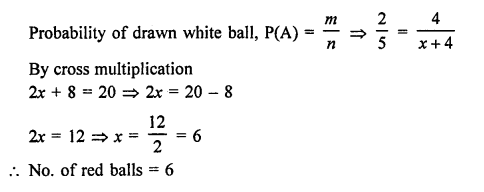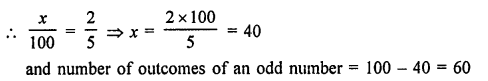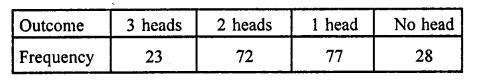• +91 9971497814
• info@interviewmaterial.com

# RD Chapter 25- Probability Ex-VSAQS Interview Questions Answers

### Related Subjects

Question 1 : Define a trial.

Answer 1 : When we perform an experiment, it is called a trial of the experiment.

Question 2 : Define an elementary event.

Answer 2 : An outcome of a trial of an experiment is called an elementary event.

Question 3 : Define an event.

Answer 3 : An event association to a random experiment is said to occur in a trial.

Question 4 : Define probability of an event.

In n trials of a random experiment if an event A happens m times, then probability of happening
of A is given by P(A) = m/n

Question 5 : A bag contains 4 white balls and some red balls. If the probability of drawing a white ball from the bag is 25, find the number of red balls in the bag

No. of white balls = 4
Let number of red balls = x
Then total number of balls (n) = 4 white + x red = (4 + x) ballsQuestion 6 : A die is thrown 100 times. If the probability of getting an even number is 25. How many times an odd number is obtained?

Total number of a die is thrown = 100
Let an even number comes x times, then probability of an even number = x/100Question 7 :
Three coins are tossed simultaneously 200 times with the following frequencies of different outcomesFind the probability of getting at most two heads.

Total number of three coins are tossed (n) = 200
Getting at the most 2 heads (m) = 72 + 77 + 28 = 177
Probability P(A) = m/n = 177/200

Question 8 : In the Q. No. 7, what is the probability of getting at least two heads?

Total number of possible events = 200
No. of events getting at the least = 2 heads (m) = 23 + 72 = 95
Probability P(A) = m/n = 95/200 = 19/40

Todays Deals### RD Chapter 25- Probability Ex-VSAQS Contributorskrishan

Name:
Email:

# Latest News# 9000 interview questions in different categories